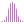#Aggregate (PQL)

Returns the aggregate for the elements in the specified list.

#### Syntax

Aggregate( <List> , OPTIONAL )

* Click on the function's arguments above for more details on the input values.

• List parameter should be a list or set of elements from an existing hierarchy in the model.
• The data point or tuple is optional, and describes the values used to generate the aggregate. If not supplied, the default measure or the query context will determine this value instead.
• For details on how to employ and use this function see the semantic calculation overview.

#### Different Function types

• This function is like the MDX aggregate function; it calculates an aggregation across a list of elements in a hierarchy or attribute.
• To use a simple sum mathematical operation on a list of numbers, use the Common library function. In the Common library, the sum is the closest to aggregate.
##### Dynamic Behavior

The aggregate function is a very powerful method that behaves differently in a variety of scenarios. Ultimately, it auto-solves numerous calculation complexities for the end user, and it should be used instead of the SUM method.

The following explains how the aggregate function behaves in a few different scenarios, depending on how the underlying granular aggregation has been defined for a column in the model:

• Sum: produces the sum of values in the list.
• Count: produces the count of values in the list.
• Max: produces the maximum value in the list.
• Min: produces the minimum value in the list.

In general, Aggregate should be used instead of Sum, because it is more often correct for most usage scenarios. See SUM for more details.

#### Examples

The following example returns the sum of expenses by product category in the product table/ dimension, using the sample demo model:

Aggregate( {AllMembers([products].[Product Category])}, ([measures].[data Expenses]) )

Below, using the sample data of the 4 product categories we can calculate the aggregate total using the above formula. 'Aggregating' the expenses for the 4 items produces 10,179,430, while the aggregate for "average expenses" produces 1019.74. This differs from the simple sum of all averages (2906) or the average of averages (726).